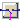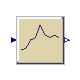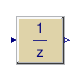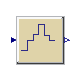## Buildings.Controls.OBC.CDL.Discrete

Package with discrete blocks

### Information

This package contains discrete control blocks with fixed sample period. Every component of this package is structured in the following way:

1. A component has continuous Real input and output signals.
2. The input signals are sampled by the given sample period defined via parameter `samplePeriod`. The first sample instant is defined by the parameter `startTime`.
3. The output signals are computed from the sampled input signals.

### Package Content

Name DescriptionFirstOrderHold First order hold of a sampled-data systemSampler Ideal sampler of a continuous signalTriggeredMax Output the maximum, absolute value of a continuous signal at trigger instantsTriggeredMovingMean Triggered discrete moving mean of an input signalTriggeredSampler Triggered sampling of continuous signalsUnitDelay Output the input signal with a unit delayZeroOrderHold Output the input signal with a zero order holdExamples Collection of models that illustrate model use and test models

##Buildings.Controls.OBC.CDL.Discrete.FirstOrderHold

First order hold of a sampled-data system### Information

Block that outputs the extrapolation through the values of the last two sampled input signals.

### Parameters

TypeNameDefaultDescription
RealsamplePeriod Sample period of component [s]

### Connectors

TypeNameDescription
input RealInputuContinuous input signal
output RealOutputyContinuous output signal

### Modelica definition

block FirstOrderHold "First order hold of a sampled-data system" parameter Real samplePeriod( final quantity="Time", final unit="s", min=1E-3) "Sample period of component"; Interfaces.RealInput u "Continuous input signal"; Interfaces.RealOutput y "Continuous output signal"; protected parameter Real t0( final quantity="Time", final unit="s", fixed=false) "First sample time instant"; output Boolean sampleTrigger "True, if sample time instant"; output Boolean firstTrigger( start=false, fixed=true) "Rising edge signals first sample instant"; Real tSample( final quantity="Time", final unit="s") "Time of sample"; Real uSample "Value of sample"; Real pre_uSample "Value of previous sample"; Real c "Slope"; initial equation t0=Buildings.Utilities.Math.Functions.round( x=integer(time/samplePeriod)*samplePeriod, n=6); pre(tSample)=t0; pre(uSample)=u; pre(pre_uSample)=u; pre(c)=0.0; equation // Declarations that are used for all discrete blocks sampleTrigger=sample( t0, samplePeriod); when sampleTrigger then firstTrigger=time <= t0+samplePeriod/2; tSample=time; uSample=u; pre_uSample=pre(uSample); c=if firstTrigger then 0 else (uSample-pre_uSample)/samplePeriod; end when; /* Use pre_uSample and pre(c) to break potential algebraic loops by an infinitesimal delay if both the continuous and the discrete part have direct feedthrough. */ y=pre_uSample+pre(c)*(time-tSample); end FirstOrderHold;

##Buildings.Controls.OBC.CDL.Discrete.Sampler

Ideal sampler of a continuous signal### Information

Block that outputs the input signal, sampled at a sampling rate defined via parameter `samplePeriod`.

### Parameters

TypeNameDefaultDescription
RealsamplePeriod Sample period of component [s]

### Connectors

TypeNameDescription
input RealInputuContinuous input signal
output RealOutputyContinuous output signal

### Modelica definition

block Sampler "Ideal sampler of a continuous signal" parameter Real samplePeriod( final quantity="Time", final unit="s", min=1E-3) "Sample period of component"; Interfaces.RealInput u "Continuous input signal"; Interfaces.RealOutput y "Continuous output signal"; protected parameter Real t0( final quantity="Time", final unit="s", fixed=false) "First sample time instant"; output Boolean sampleTrigger "True, if sample time instant"; output Boolean firstTrigger( start=false, fixed=true) "Rising edge signals first sample instant"; initial equation t0=Buildings.Utilities.Math.Functions.round( x=integer(time/samplePeriod)*samplePeriod, n=6); equation // Declarations that are used for all discrete blocks sampleTrigger=sample( t0, samplePeriod); when sampleTrigger then firstTrigger=time <= t0+samplePeriod/2; end when; // Declarations specific to this type of discrete block when {sampleTrigger,initial()} then y=u; end when; end Sampler;

##Buildings.Controls.OBC.CDL.Discrete.TriggeredMax

Output the maximum, absolute value of a continuous signal at trigger instants### Information

Block that outputs the input signal whenever the trigger input signal is rising (i.e., trigger changes to `true`). The maximum, absolute value of the input signal at the sampling point is provided as the output signal.

### Connectors

TypeNameDescription
input RealInputuConnector with a Real input signal
input BooleanInputtriggerConnector for trigger
output RealOutputyConnector with a Real output signal

### Modelica definition

block TriggeredMax "Output the maximum, absolute value of a continuous signal at trigger instants" Interfaces.RealInput u "Connector with a Real input signal"; Interfaces.BooleanInput trigger "Connector for trigger"; Interfaces.RealOutput y "Connector with a Real output signal"; initial equation y=u; equation when trigger then y=max( pre(y), abs(u)); end when; end TriggeredMax;

##Buildings.Controls.OBC.CDL.Discrete.TriggeredMovingMean

Triggered discrete moving mean of an input signal### Information

Block that outputs the triggered moving mean value of an input signal.

At the start of the simulation, and whenever the trigger signal is rising (i.e., the trigger changes to `true`), the block samples the input, computes the moving mean value over the past `n` samples, and produces this value at its output `y`.

### Parameters

TypeNameDefaultDescription
Integern Number of samples over which the input is averaged

### Connectors

TypeNameDescription
input RealInputuContinuous input signal
input BooleanInputtriggerBoolean signal that triggers the block
output RealOutputyDiscrete averaged signal

### Modelica definition

block TriggeredMovingMean "Triggered discrete moving mean of an input signal" parameter Integer n( min=1) "Number of samples over which the input is averaged"; Interfaces.RealInput u "Continuous input signal"; Interfaces.BooleanInput trigger "Boolean signal that triggers the block"; Interfaces.RealOutput y "Discrete averaged signal"; protected Integer iSample( start=0, fixed=true) "Sample numbering in the calculation"; Integer counter( start=0, fixed=true) "Number of samples used for averaging calculation"; Integer index( start=0, fixed=true) "Index of the vector ySample"; discrete Real ySample[n]( start=zeros(n), each fixed=true) "Vector of samples to be averaged"; equation when {initial(),trigger} then index=mod( pre(iSample), n)+1; ySample={ if (i == index) then u else pre(ySample[i]) for i in 1:n}; counter= if pre(counter) == n then n else pre(counter)+1; y=sum(ySample)/counter; iSample=pre(iSample)+1; end when; end TriggeredMovingMean;

##Buildings.Controls.OBC.CDL.Discrete.TriggeredSampler

Triggered sampling of continuous signals### Information

Samples the continuous input signal whenever the trigger input signal is rising (i.e., trigger changes from `false` to `true`) and provides the sampled input signal as output. Before the first sampling, the output signal is equal to the initial value defined via parameter `y_start`.

### Parameters

TypeNameDefaultDescription
Realy_start0Initial value of output signal

### Connectors

TypeNameDescription
input RealInputuConnector with a Real input signal
input BooleanInputtriggerSignal that triggers the sampler
output RealOutputyConnector with a Real output signal

### Modelica definition

block TriggeredSampler "Triggered sampling of continuous signals" parameter Real y_start=0 "Initial value of output signal"; Interfaces.RealInput u "Connector with a Real input signal"; Interfaces.BooleanInput trigger "Signal that triggers the sampler"; Interfaces.RealOutput y "Connector with a Real output signal"; initial equation y=y_start; equation when trigger then y=u; end when; end TriggeredSampler;

##Buildings.Controls.OBC.CDL.Discrete.UnitDelay

Output the input signal with a unit delay### Information

Block that outputs the input signal with a unit delay:

```          1
y = --- * u
z
```

that is, the output signal `y` is the input signal `u` of the previous sample instant. Before the second sample instant, the output `y` is identical to parameter `y_start`.

### Parameters

TypeNameDefaultDescription
RealsamplePeriod Sample period of component [s]
Realy_start0Initial value of output signal

### Connectors

TypeNameDescription
input RealInputuContinuous input signal
output RealOutputyContinuous output signal

### Modelica definition

block UnitDelay "Output the input signal with a unit delay" parameter Real samplePeriod( final quantity="Time", final unit="s", min=1E-3) "Sample period of component"; parameter Real y_start=0 "Initial value of output signal"; Interfaces.RealInput u "Continuous input signal"; Interfaces.RealOutput y "Continuous output signal"; protected parameter Real t0( final quantity="Time", final unit="s", fixed=false) "First sample time instant"; output Boolean sampleTrigger "True, if sample time instant"; initial equation t0=Buildings.Utilities.Math.Functions.round( x=integer(time/samplePeriod)*samplePeriod, n=6); y=y_start; equation // Declarations that are used for all discrete blocks sampleTrigger=sample( t0, samplePeriod); when sampleTrigger then y=pre(u); end when; end UnitDelay;

##Buildings.Controls.OBC.CDL.Discrete.ZeroOrderHold

Output the input signal with a zero order hold### Information

Block that outputs the sampled input signal at sample time instants. The output signal is held at the value of the last sample instant during the sample points. At initial time, the block feeds the input directly to the output.

### Parameters

TypeNameDefaultDescription
RealsamplePeriod Sample period of component [s]

### Connectors

TypeNameDescription
input RealInputuContinuous input signal
output RealOutputyContinuous output signal

### Modelica definition

block ZeroOrderHold "Output the input signal with a zero order hold" parameter Real samplePeriod( final quantity="Time", final unit="s", min=1E-3) "Sample period of component"; Interfaces.RealInput u "Continuous input signal"; Interfaces.RealOutput y "Continuous output signal"; protected parameter Real t0( final quantity="Time", final unit="s", fixed=false) "First sample time instant"; output Real ySample( fixed=true, start=0) "Sampled value of input"; output Boolean sampleTrigger "True, if sample time instant"; output Boolean firstTrigger( start=false, fixed=true) "Rising edge signals first sample instant"; initial equation t0=Buildings.Utilities.Math.Functions.round( x=integer(time/samplePeriod)*samplePeriod, n=6); equation // Declarations that are used for all discrete blocks sampleTrigger=sample( t0, samplePeriod); when sampleTrigger then firstTrigger=time <= t0+samplePeriod/2; end when; // Declarations specific to this type of discrete block when {sampleTrigger,initial()} then ySample=u; end when; /* Define y=ySample with an infinitesimal delay to break potential algebraic loops if both the continuous and the discrete part have direct feedthrough */ y=pre(ySample); end ZeroOrderHold;